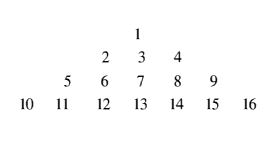[latexpage]

# NumbersIf the pattern shown is continued, what number will appear below 400?

### Solve our previous ‘questions of the day’

1.800

1.Sorry, its 440,
Number of digits in each row is odd number.(1,3,5,7….)
And last digit in each row is sum of number of digits in all previous rows (16=1+3+5+7). Therefore, using AP if sum is 400, n=20. And the number of digits in a row is the summision of (n+n-1). Therefore, number of digits in 20th row is 39 and the number below 400 will be +40(39+1) i.e. 440(400+40)

1.Correct!

2.No shown below 400 is 21^2 -1

= 440 (ans)
reason- if you observe the last digit of every row you will find the square of numbers from 1…..n
then 400 is sq of 20 hence 20 will be in right corner
and in the next row 21^2 will be in right corner
therefore 440 is the ans

1.Perfect !

3.Yamin.yaqoob

1.Correct!

4.Abhishek Tandon

440

Ending term of each line is a square so 400 line will have 39 terms next line will have 41 terms so the 40th term of that line is 400+40=440 which is below 400.

5.440

6.Gaurav raj

20*20=400
so 21*21=441
441-1=440

7.Dhruv Agarwal

440

8.Aayushi Lal

21^2-1=440

• Nice instruction, thanks

By LouisHayes , 3 months ago

• In a test match in cricket, the scores of Rohit and Vir...

By nhuadovan , 11 months ago

• The 111th digit of the series 149162536496481........ i...

By nhuadovan , 11 months ago

• how many perfect squares of natural numbers between 300...

By nhuadovan , 11 months ago

• Find the number of ways to distribute 2n different ball...

By nhuadovan , 11 months ago

• A cube is divided into two parts by a plane passing thr...

By nhuadovan , 11 months ago

• @utkarsh-garg25 how

By traciblack , 11 months ago

• Thank you for sharing the list ... i will try to read o...

By Anonymous , 1 year ago

• The collection of the questions are really great it wil...

By Anonymous , 2 years ago

• Mark bought 45 grinders for Rs. 2215, while carrying h...

By Anonymous , 2 years ago

• Great thread ,i tried to do these but not able to do..t...

By Anonymous , 2 years ago